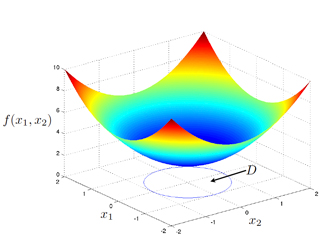10.34 | Fall 2015 | Graduate

# Numerical Methods Applied to Chemical Engineering

#### Course Description

Numerical methods for solving problems arising in heat and mass transfer, fluid mechanics, chemical reaction engineering, and molecular simulation. Topics: Numerical linear algebra, solution of nonlinear algebraic equations and ordinary differential equations, solution of partial differential equations (e.g. …

## Course Info

##### Learning Resource Types
Lecture Notes
Programming Assignments
Lecture Videos
Exams with SolutionsOptimization of a convex function of two variables. (Image courtesy of James W. Swan)